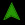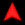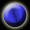<< INTRODUCTION >> << OVERVIEW >> << AUDIO >> << GAMEPLAY >> << VIDEO >> << IMPROVEMENTS >> << ZBT RAMS >> << TIPS AND TRICKS >> << NES INPUT FSM >> << TOOLS >> Appendix: NES Input FSM ```/* NINTENDO CONTROLLER INPUT FSM The NES controller connects to the system via 5 wires. 2 wires are power and ground The others are latch, pulse and data. Latch and pulse are signals from the FSM to the controller. Data is a signal from the controller to the FSM. The data read process starts on the gameclock signal. The data protocol (exactly as used by Nintento itself) is as follows: 1. FSM sends latch signal high for 12 us to controller (LATCH) 2. "A" button data (high or low) is ready on data line (READ_A) 3. FSM waits 6 us (WAIT) 4. FSM sends pulse signal high for 6 us to controller (PULSE) 5. "B" button data (high or low) is ready on data line (READ_B) 6. Repeat steps 3-5 for (in order): select button (READ_SELECT) start button (READ_START) up button (READ_UP) down button (READ_DOWN) left button (READ_LEFT) right button (READ_RIGHT) For each WAIT-PULSE sequence, the return read state is stored in returnstate. Output data is registered with the 27 MHz clock before passing it on. */ module gameinput(clock, gameclock, reset, latch, pulse, data, plyr_input); input clock, gameclock, reset, data; output latch, pulse; reg latch, latch1, pulse, pulse1, data1; output [7:0] plyr_input; reg left, right, up, down, A, B, select, start; reg left1, right1, up1, down1, A1, B1, select1, start1; assign plyr_input = {left, right, up, down, A, B, select, start}; reg [3:0] state, nextstate, returnstate, nextreturnstate; reg [11:0] count, nextcount; parameter INIT = 0; parameter IDLE = 1; parameter LATCH = 2; parameter WAIT = 3; parameter PULSE = 4; parameter READ_A = 5; parameter READ_B = 6; parameter READ_SEL = 7; parameter READ_STRT = 8; parameter READ_UP = 9; parameter READ_DOWN = 10; parameter READ_LEFT = 11; parameter READ_RIGHT = 12; parameter TWELVE_US = 12'h144; //count for 12 us on a 27 MHz clock parameter SIX_US = 12'h0A2; //count for 6 us on a 27 MHz clock always @ (posedge clock) begin if (!reset) begin state <= INIT; returnstate <= INIT; count <= 0; end else begin state <= nextstate; returnstate <= nextreturnstate; count <= nextcount; end data1 <= data; latch <= latch1; pulse <= pulse1; left <= left1; right <= right1; up <= up1; down <= down1; A <= A1; B <= B1; select <= select1; start <= start1; end always @ (state or returnstate or count or gameclock or data1) begin //defaults nextstate = state; nextreturnstate = returnstate; nextcount = count; latch1 = latch; pulse1 = pulse; left1 = left; right1 = right; up1 = up; down1 = down; A1 = A; B1 = B; select1 = select; start1 = start; case (state) INIT: begin nextstate = IDLE; nextcount = 0; end IDLE: begin nextcount = 0; //get input at input rate specified by game clock if (gameclock) nextstate = LATCH; end LATCH: begin //latch 12 us, then go to read A latch1 = 1; if (count == TWELVE_US) begin nextcount = 0; latch1 = 0; nextstate = READ_A; end else nextcount = count + 1; end WAIT: begin //wait 6 us, then go to pulse if (count == SIX_US) begin nextcount = 0; nextstate = PULSE; end else nextcount = count + 1; end PULSE: begin //pulse 6 us, then go to returnstate and read data pulse1 = 1; if (count == SIX_US) begin nextcount = 0; pulse1 = 0; nextstate = returnstate; end else nextcount = count + 1; end READ_A: begin A1 = ~data1; nextreturnstate = READ_B; nextstate = WAIT; end READ_B: begin B1 = ~data1; nextreturnstate = READ_SEL; nextstate = WAIT; end READ_SEL: begin select1 = ~data1; nextreturnstate = READ_STRT; nextstate = WAIT; end READ_STRT: begin start1 = ~data1; nextreturnstate = READ_UP; nextstate = WAIT; end READ_UP: begin up1 = ~data1; nextreturnstate = READ_DOWN; nextstate = WAIT; end READ_DOWN: begin down1 = ~data1; nextreturnstate = READ_LEFT; nextstate = WAIT; end READ_LEFT: begin left1 = ~data1; nextreturnstate = READ_RIGHT; nextstate = WAIT; end READ_RIGHT: begin right1 = ~data1; nextstate = IDLE; end endcase end endmodule ```